# Quick Summary (Probability)University is starting for some students who took A’levels in 2016. And, one of my ex-students told me to share/ summarise the things to know for probability at University level. Hopefully this helps. H2 Further Mathematics Students will find some of these helpful.

Supposeis a random variable which can takes values.is a discrete r.v. isis countable.is the probability of a value ofand is called the probability mass function.is a continuous r.v. isis uncountable.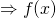is the probability density function and can be thought of as the probability of a value.

Probability Mass Function

For a discrete r.v. the probability mass function (PMF) is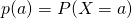, where.

Probability Density Function

If.

And strictly speaking,.

Intuitively,.

Properties of Distributions

For discrete r.v.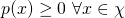..

For continuous r.v...

Cumulative Distribution Function

For discrete r.v., the Cumulative Distribution Function (CDF) is.

For continuous r.v., the CDF is.

Expected Value

For a discrete r.v. X, the expected value is.

For a continuous r.v. X, the expected value is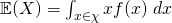.

If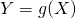, then

For a discrete r.v. X,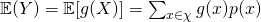.

For a continuous r.v. X,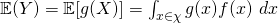.

Properties of Expectation

For random variablesand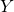and constants, the expected value has the following properties (applicable to both discrete and continuous r.v.s)Realisations of, denoted by, may be larger or smaller than,

If you observed many realisations of,is roughly an average of the values you would observe.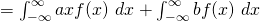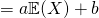Variance

Generally speaking, variance is defined asIfis discrete: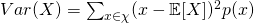Ifis continuous:Using the properties of expectations, we can show.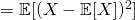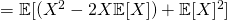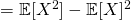Standard Deviation

The standard deviation is defined asCovariance

For two random variablesand, the covariance is generally defined asNote that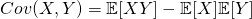Properties of Variance

Given random variablesand, and constants,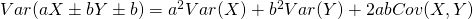This proof for the above can be done using definitions of expectations and variance.

Properties of Covariance

Given random variablesandand constants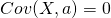Correlation

Correlation is defined as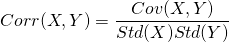It is clear the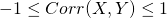.

The properties of correlations of sums of random variables follow from those of covariance and standard deviations above.

Not readable? Change text.